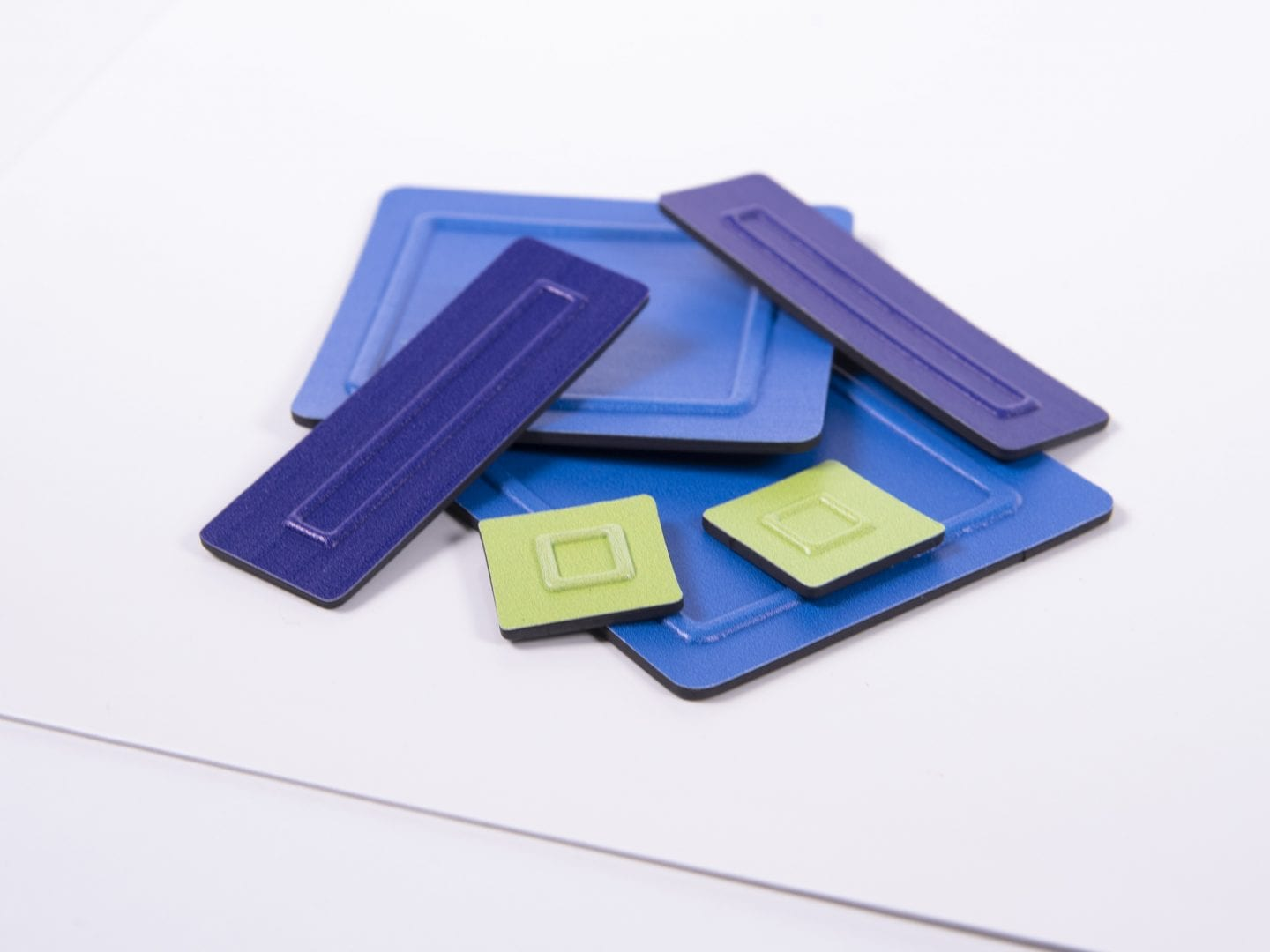#Solving Equations Using Tactile Algebra Tiles

Are you looking for an easy-to-understand hands-on way to explain algebra concepts to your child? Tactile Algebra Tiles can help! It provides students with concrete models for approaching abstract math concepts and procedures. Wondering how? Grab your Tactile Algebra Tiles and we’ll show you how!

Let’s get started. Open the box of a Tactile Algebra Tiles kit and take out one steel board. We are going to use the tiles listed below. Get them from the tile bags and place them on half of the board.

• Ten of the small lime square tiles with a tactile square symbol at the center. These represent the numerical value +1.
• Ten of the small red square tiles with a raised dot at the center. These represent the numerical value -1.
• Two of the violet rectangle tiles with a tactile rectangular symbol at the center. These represent the numerical value +x.
• One Long, narrow, black separation bar.

We’ll start with the equation x + 3 = 1 as an example. When modeling an equation using tiles, we can use a black separation bar to separate the two sides of the equation. We can see it as the “=” sign. Put the bar in the middle of half of your board. Place one violet rectangle (one positive x) and three small lime squares (three positive 1s) to the left of the bar, and one small lime square (one positive 1) to the right of the bar. Now, from left to right, the tiles represent: x + 3 = 1.

To solve this equation, we need to remove the number 3 from the left side. In order to do so, add number -3 to the left side. That is to add three small red squares to the left side of the bar. Of course, to keep the balance of the equation, the exact same action needs to be done to the right side of the bar. So, also add three red squares to the right side. Now, the tiles represent: x + 3 – 3 = 1 – 3.

Next, do some simple addition on either side of the equation. On the left side, the sum of 3 – 3 is 0, and therefore those six tiles can be removed from the equation. Zero means nothing, correct? On the right side, the sum of one positive 1 and one of the three negative 1s “1 – 1” is 0. Therefore those two tiles can be removed. The other two small red squares remain.

Now, read from left to right, the tiles represent: x = -2. That is the answer of this equation. Not to difficult, right?

Let’s see another example: 2x – 1 = 5.

Similarly, the first step is to use the tiles to represent the equation. Put the separation bar in the middle. Then place two violet rectangles (two positive xs) and one small red square (one -1) to the left of the bar, and five small lime squares (five positive 1s) to the right of the bar.

Add one small lime square (one positive 1) to either side of the bar. On the left side, because the sum of the newly added small lime square and the original small red square is 0, both tiles can be removed. Now, the equation becomes: 2x = 6.

Next, on either side of the bar, separate the tiles into two groups. Two violet rectangles should now be on the left side of the bar and two groups of three small lime squares should be on the right side of the bar.

Last, divide either side of the equation by 2. That is 2x / 2 = x on the left and 6 / 2 = 3 on the right. To reflect the division results, remove one group of tiles from each side. The answer to this equation is x = 3.

Got it? Here are more problems for practice. Get more tiles from the box as needed.

• x – 2 = 2 (answer: x = 4)
• x + 1 = -4 (answer: x = -5)
• 2x + 7 = 1 (answer: x = -3)
• 3x – 1 = 5 (answer: x = 2)

Solving equations using physical pieces. Isn’t that interesting? Children have diverse learning styles. For those hands-on learners, Tactile Algebra Tiles may do the trick. Besides solving equations, this kit can also be used by students ranging from early elementary to high school for counting, sorting, basic arithmetic calculations, simplifying expressions, and adding, subtracting, multiplying, and factoring polynomials. You can find more instructions on its use in the product guidebook.